Home > CC2 > Chapter Ch1 > Lesson 1.1.4 > Problem1-37

1-37.

As you can tell from the examples of the number lines below, not all number lines change by one unit from mark to mark. Copy these number lines onto your paper and fill in the missing numbers. Homework Help ✎

1.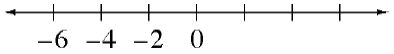Find the difference between two marks on the number line.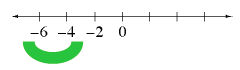The numbers grow by $2$ units. Copy the number line on your paper and label the other marks.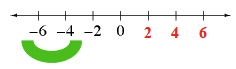1.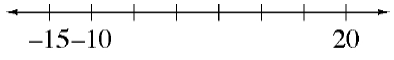Find the difference between two marks on the number line.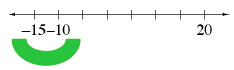The numbers grow by $5$ units. Copy the number line on your paper and label the other marks.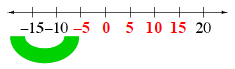1.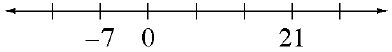See (a) and (b).

1.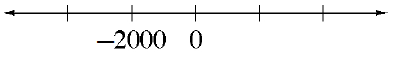See (a) and (b).